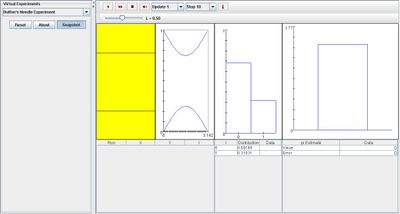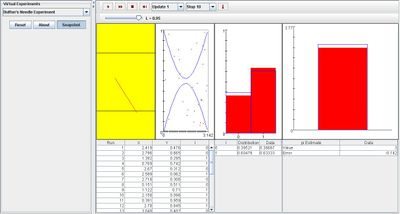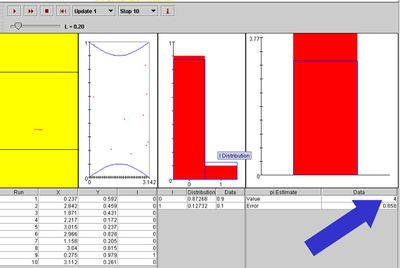# SOCR EduMaterials Activities BuffonNeedleExperiment

## Description

The Buffon’s Needle Experiment involves dropping a needle on hardwood floor with floorboards of width 1. The experiment is shown graphically in the first graph box (see image below).

The angle X of the needle relative to the floorboard cracks and the distance Y from the center of the needle to the lower crack are recorded in the first table on each update. In the distribution table which is the distribution table, the values of the density of I, empirical density of I are recorded, where variable I indicates the event that the needle crosses a crack. The values of pi ($$\pi$$) and Buffon’s estimate of pi are recorded in the last table.

Each point (X,Y) is shown as a red dot in the scatter plot. The density of I is shown in blue and the empirical density of I is shown in red in the distribution graph. In the last graph, pi is shown in blue and the Buffon’s estimate of pi is shown in red.

The parameter is the needle length L which can be modified with the scroll bar.

## Goal

To provide a simulation that describes the probability of random events and to justify hypotheses and estimations. This demonstration should provide a better understanding and knowledge regarding calculus based probability.

## Experiments

Go to the SOCR Experiments and select the Buffon Needle Experiment from the drop-down list of experiments on the top left. The image below shows the initial view of this experiment:When pressing the play button, one trial will be executed and recorded in the distribution table below. The fast forward button symbolizes the nth number of trials to be executed each time. The stop button ceases any activity and is helpful when the experimenter chooses “continuous,” indicating an infinite number of events. The fourth button will reset the entire experiment, deleting all previous information and data collected. The “update” scroll indicates nth number of trials (1, 10, 100, or 1000) performed when selecting the fast forward button and the “stop” scroll indicates the maximum number of trials in the experiment.

With the initial conditions, the distribution graph displays a lower value, I, in which the needle has a lesser chance of crossing a crack (1=success, 0=failure). After many trials, the empirical density graphs (red) should look similar to the density graph (blue), and the Buffon’s pi estimate (red) should look similar to the pi graph (blue).

When L increases, the probability that the needle will cross a crack also increases. This is shown in the image below:When L decreases, the probability that the needle will cross a crack also decreases. This is shown in the image below:## Buffon's needle experiment and estimation of the constant $$\pi$$

Suppose the length of the needle is $$0\le L\le 1$$ and the distance between the lines is set to 1. When the needle is dropped, there are two important measurements we make to determine if the needle crosses any of the the grid-lines - these are the angle at which the needle falls ($$\alpha$$ ) and the distance between the center of the needle to the closest line (d). Note that $$0\le \alpha \le \pi$$ and $$0\le d \le {1 \over 2}$$. The needle will cross a grid-line if $$d \le {L \over 2} \sin(\alpha)$$. What are the odds of this happening?

The distribution of the pairs of ($$\alpha, d)$$ for which this inequality is satisfied is presented in the graph below. The shaded region on the right represents the situation when the needle crosses a grid-line (i.e., $$d \le {L \over 2} \sin(\alpha)$$). Therefore, the probability of an needle-line intersection is determined by the ratio of the shaded area to the area of the enclosing rectangle. The area of the shaded area is computed by $$\int_{0}^{\pi} {{L \over 2} \sin(\alpha) d \alpha} = L$$ and the area of the rectangle is $${1 \over 2}\pi$$. Then, the odds of a needle-line intersection are $${ L \over {\pi\over 2}}={2 L \over \pi}$$ (if $$L=1$$, then $${2 \over \pi}\approx 0.63662$$). In practice, to estimate $$\pi$$ from a number of needle drops (N), we take the reciprocal of the sample odds-of-intersection. This allows us to empirically estimate $$\pi \approx {1 \over odds-of-intersection} = {2 L \over { Number-Of-Intersections \over N}}.$$For example, suppose $$N=10$$, $$L=0.2$$ and we observe 1 needle-line crossing in the 10 trials. Then our estimate of $$\pi \approx { 2 \over {1 \over 10\times 0.2}} = 4$$, as shown on the image below.In general, when the grid-line tiles are of size S, the formulas slightly change as follows: area of the rectangle is $${S \over 2}\pi$$ and the odds of a needle-line intersection is $${ L \over {S \times \pi\over 2}}={2 L \over S \times \pi}$$. Estimation of $$\pi$$ from a number of needle drops (N) is then obtained by $$\pi \approx {1 \over odds-of-intersection} = {2 L \over S} \times {1 \over {Number-Of-Intersections \over N}}.$$

## Applications

The Buffon’s Needle Experiment is a simulation that is applicable for anyone to describe calculus based probability events. This java applet may be used for many different types of activities.

One example may be for throwing darts onto a dart board. When using a dart made of a fixed material, a shorter length dart will have lighter weight so it may be easier to control the dart, thus there will be a higher chance that the dart will land within the target. But a longer dart may experience more air resistance and have a heavier weight, causing the probability to land within the target to decrease.